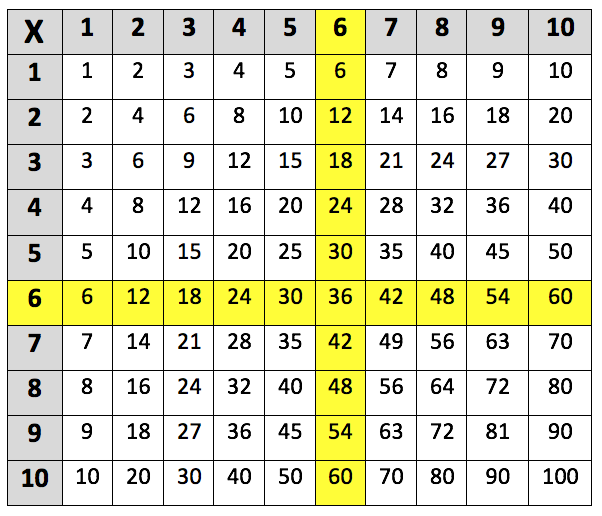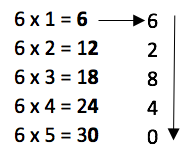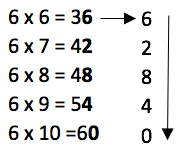Learning the 6 times table. The 6 times table up to 6 x 10 is highlighted in the multiplication grid below:This is the 6 times table up to 6 x 12:
6 x 1 = 6
6 x 2 = 12
6 x 3 = 18
6 x 4 = 24
6 x 5 = 30
6 x 6 = 36
6 x 7 = 42
6 x 8 = 48
6 x 9 = 54
6 x 10 = 60
6 x 11 = 66
6 x 12 = 72

## When do children learn the 6 times table?

During Year 4, children will learn the 6, 7, 9, 11 and 12 times tables. By the end of Year 4, children should have been taught all the times tables up to the 12 times table. Teachers will start giving children harder multiplications to complete, these will include multiplying three-digit numbers by a one-digit number (such as 150 x 3 = 450).

Children will also be taught division facts for the 6 times table, such as:

6 ÷ 6 = 1 and 12 ÷ 6 = 2

## How will children learn the 6 times table?

Teachers will try different ways of encouraging children to learn their times tables, it may be useful to talk to your child’s teacher and ask them how to best support your child with their times tables learning at home.

Teachers should be making sure that children get plenty of practice answering times table questions. One way they might do this is by giving children a worksheet which asks them to match up a multiplication question with the correct answer. For example:

Use a coloured pencil to shade each multiplication star and match it with the correct answer circle.## How to help children with the 6 times table?

It can be helpful for children to learn that every number in the 6 times table is an even number and that the final digits repeat themselves in a pattern. For example:Children could then approach the 6 times tables by learning it in two halves.

If children are confident with their 3 times table, it is useful to know that the digits of a multiple of 6 will add up to be a multiple of 3. Knowing this makes it easy for children to work out whether some even numbers are multiples of 6. For example, the number 44 is not a multiple of 6 because 4 + 4 = 8, which is not a multiple of 3.

## How does Learning street help children with the 6 times table?

Repetition is key to learning your 6 times table, and in the Learning Street 8 year old courses, there is a constant focus on always practising times tables as this is the best way to improve. This is through times tables tests (to improve accuracy), tables races (to improve time), mental mathematics, maths problem solving and other fun activities such as mystery pictures.

Without a good knowledge of times tables, it can lead to problems in SATs, GCSEs and A Levels.

## Our Courses

Click through to review the courses we have available

##### Get Started

Learning Street structured courses make home study easier and more successful. There's no need to wonder which books to buy or what to do next. Everything is planned for you.

• Proven to deliver excellent results
• Used by families, private tutors and schools
• Fully planned and structured